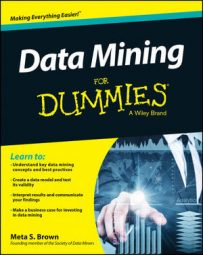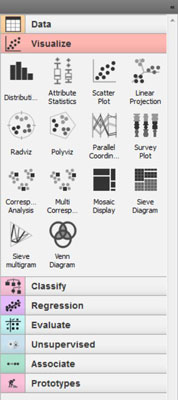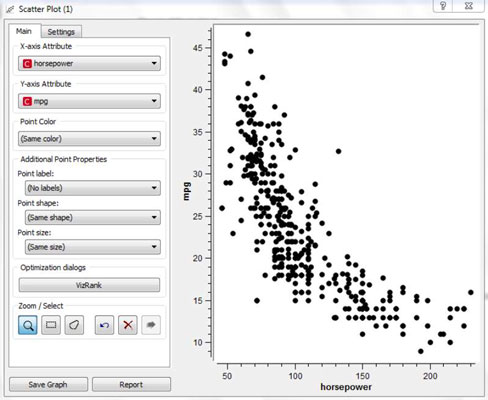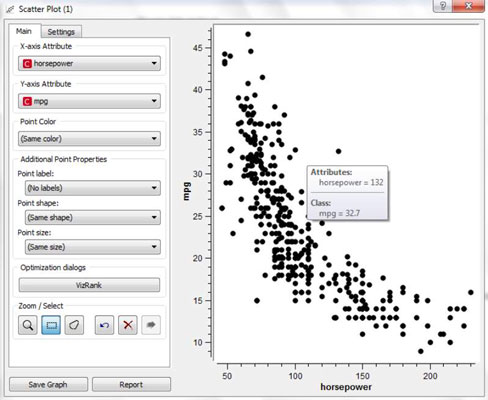##### Data Mining For DummiesThe first step toward predictive modeling is relating variables to one another. A simple, remarkable tool for that is the scatterplot. It’s used to relate one continuous measure to another. Data miners sometimes stretch the rules and use it with categorical variables as well.

The horizontal (x) axis of the plot represents values of one variable; the vertical axis (y) represents a second variable. You may not have a sense of which variable is independent and which is dependent for every pair of variables.

If you do, the independent variable should be on the horizontal axis. Each point on the plot represents the coordinates, the pair of values for the two variables within a single case. (These pairs are sometimes called xy pairs).

Find your scatterplot tool and set up a basic scatterplot tool by selecting two variables to use. The following figure shows this tool on the menu of Orange; the location for the tool varies by product.The example in the next image shows an interactive display; the scatterplot appears immediately. In another tool, you might need additional steps to execute and create the chart.The scatterplot example relates auto mileage to engine horsepower. Low horsepower is associated with high mileage, and the higher the horsepower, the lower the mileage. You can easily see this pattern in the data. You might notice a shape, not linear but somewhat curved. This could provide hints about what model types to try later.

Data-mining applications often have some interactive features in graph displays. For example, the next figure shows that hovering your mouse over a point reveals the exact values of the two variables for that point. This is easier than trying to read the values from the axes!